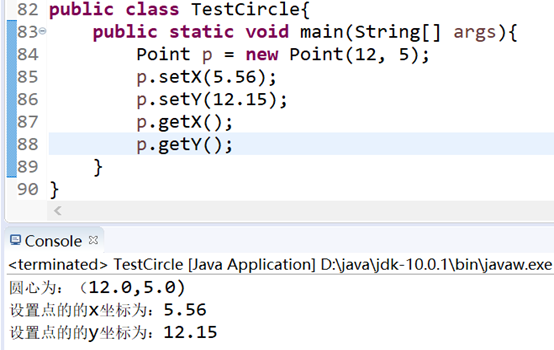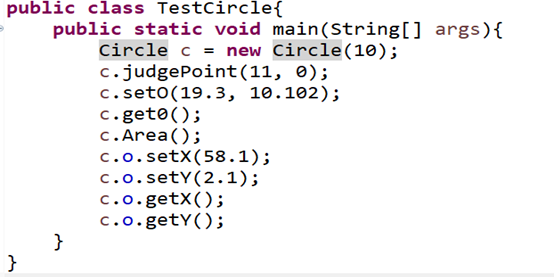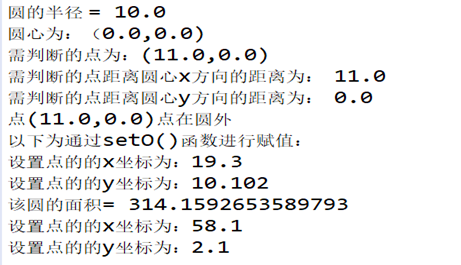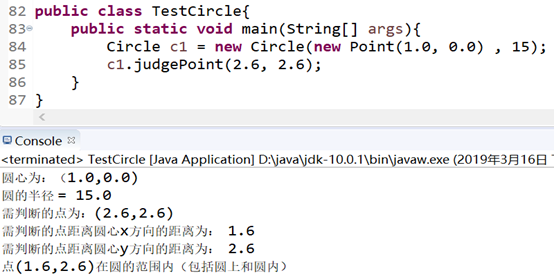• 判断点是否在圆内思路相似，将P(x,y)的x代入此椭圆方程（x-x1)^2/A^2+(y-y1)^2/B^2=1;得到解（2个，1个，无解）。无解，当然不在椭圆内。有解，P点的y值是否[Min（解集合）,Max（解集合）]之中，若在椭圆...
跟判断点是否在圆内思路相似，将P(x,y)的x代入此椭圆方程（x-x1)^2/A^2+(y-y1)^2/B^2=1;得到解（2个，1个，无解）。无解，当然不在椭圆内。有解，P点的y值是否在[Min（解集合）,Max（解集合）]之中，若在，在椭圆范围之内；否则，在椭圆之外。

如果X X1 A B 都已知的话，那么就是和1进行比较，如果小于1的话 就在椭圆内部，如果大于1的话，就在椭圆外部。

展开全文• 判断点是否在圆上(java) package zuoye; class Point{ double x; double y; //设置点的x坐标 public void setX(double x){ this.x = x; } //设置点的y坐标 public void setY(double y){ ...
判断点是否在圆上(java)
package zuoye;

class Point{
double x;
double y;

//设置点的x坐标
public void setX(double x){
this.x = x;
}

//设置点的y坐标
public void setY(double y){
this.y = y;
}

//获取点的x坐标
public double getX(){
System.out.println("设置点的的x坐标为：" + x);
return x;
}

//获取点的y坐标
public double getY(){
System.out.println("设置点的的y坐标为：" + y);
return y;
}
Point(double x,double y){
this.x = x;
this.y = y;
System.out.println("圆心为：（" + x + "," + y +")");
}
}

class Circle{
Point o;

//求圆的面积
public double Area(){
}
o = new Point(0.0,0.0);
}
o = p;

}
public void setO(double x,double y){
System.out.println("以下为通过setO()函数进行赋值：");
o.setX(x);
o.setY(y);
}
public void get0(){
o.getX();
o.getY();
}

//判断点是否在圆上
public Boolean judgePoint(double a, double b){
System.out.println("需判断的点为：(" + a + "," + b + ")");
double x = Math.abs(a - o.x);        //需判断的点距离圆心x方向的距离
System.out.println("需判断的点距离圆心x方向的距离为：  " + x);
double y = Math.abs(b - o.y);        //需判断的点距离圆心y方向的距离
System.out.println("需判断的点距离圆心y方向的距离为：  " + y);
if(x * x + y * y < radius * radius){     //此处的x为设置点和圆心的距离，y也是如此
System.out.println("点(" + x + "," + y + ")在圆的范围内（包括圆上和圆内）");
return true;
}else{
System.out.println("点(" + x + "," + y + ")点在圆外");
return false;
}
}
}

public class TestCircle{
public static void main(String[] args){
Point p = new Point(12, 5);
p.setX(5.56);
p.setY(12.15);
p.getX();
p.getY();

Circle c = new Circle(10);
c.judgePoint(11, 0);
c.setO(19.3, 10.102);
c.get0();
c.Area();
c.o.setX(58.1);  //通过o调用Point类中的setX()函数
c.o.setY(2.1);   //通过o调用Point类中的setY()函数
c.o.getX();      //通过o调用Point类中的getX()函数
c.o.getY();      //通过o调用Point类中的getY()函数

Circle c1 = new Circle(new Point(1.0, 0.0) , 15);
c1.judgePoint(2.6, 2.6);
}
}


对Point类中的方法进行测试的代码和截图如下：对Circle类中的单参数构造方法以及其他方法进行测试代码和截图如下：对Circle类中的双参数构造方法进行测试代码和截图如下：展开全文java
• //判断点在圆内 private boolean inOval(PointBean point,PointBean centerPoint) { double v = Math.pow(centerPoint.x-point.x, 2) / Math.pow(a, 2) + Math.pow(centerPoint.y-point.y, 2) / Math.pow(b, 2); r...
//判断点在圆内
private boolean inOval(PointBean point,PointBean centerPoint) {
double v = Math.pow(centerPoint.x-point.x, 2) / Math.pow(a, 2) + Math.pow(centerPoint.y-point.y, 2) / Math.pow(b, 2);
return v < 1;
}
展开全文• 前面已经说了，素域Fp椭圆曲线E由（仿射）方程y2＝x3＋ax＋b决定，所以Jacobi射影P＝(X,Y,Z)在不在曲线就看这个是不是满足射影方程y2＝x3＋axz4＋bz6。带入P分别计算方程左右两边再做比较即可。 同样地...
判断一个点在曲线上的理论依据是什么呢？前面已经说了，素域Fp上的椭圆曲线E由（仿射）方程y2＝x3＋ax＋b决定，所以Jacobi射影点P＝(X,Y,Z)在不在曲线上就看这个点是不是满足射影方程y2＝x3＋axz4＋bz6。带入P点分别计算方程左右两边再做比较即可。

同样地，如果P点的Z分量为1，计算量就可以大大降低，这就是在点的定义中加入参数Z_is_one的好处。

下面来解释判断点是否在曲线上的函数。

───────────────────────────────────────

int EC_POINT_is_on_curve(const EC_GROUP *group, const EC_POINT *point)

功能：    判断点是否在曲线上

输入：    group，point

输出：    －

返回：    1【在曲线上】 or 0【不在曲线上】 or －1【出错】

出处：    ec_lib.c

调用：     ▼   int ec_GFp_simple_is_on_curve(const EC_GROUP *group, const EC_POINT *point)

───────────────────────────────────────

展开全文openssl百度地图
• * 判读一个是否多边形内 返回boolean true存在 false存在 * @param point * @param polygon * @return */ public boolean checkWithJdkPolygon(Point2D.Double point, List<Point2D.Double> ...
• 平面，如果已知△P0P1P2\triangle P_0 P_1 P_2的三个顶点坐标P0(x0,y0), P1(x1,y1), P2(x2,y2)P_0(x_0, y_0),\space P_1(x_1,y_1),\space P_2(x_2,y_2)和另一点PP的坐标(x,y)(x,y)，要判断点P是否△P0P1P2\...几何算法 向量积
• 点在多边形内常用的算法就是使用射线法，作为笔记直接代码： /** * 判断一个是否多边形内部 * @param points 多边形坐标集合 * @param testPoint 测试坐标 * 返回true为真，false为假 * */ ...
• //定义一个Point类，其属性包括的坐标，提供计算 ...//判断两个是否相交并输出结果 #include&lt;iostream&gt; using namespace std; class Point { public: void setPointt(int _x1,...
• 判断一个是否指定的内，c sharpe codec#
• **输出格式要求：“该点在圆内\n” “该点不在圆内\n” “该点在圆上\n” 程序示例运行如下： 请输入的圆心坐标：3.5,4.5 请输入的半径：6 请输入要判断的点的坐标(x,y)：5.5,7.8 该点在圆内 【程序如下】 #ic语言
• # 到两个端点所确定的直线的距离　classic formula is: # # d = [(x2-x1)*(y1-y)-(x1-x)*(y2-y1)] / sqrt((x2-x1)**2 + (y2-y1)**2) # xD = (x2 - x1) yD = (y2 - y1) detaX = x1 - X detaY = y1 - Y...
• * @fileoverview GeoUtils类提供若干几何算法，用来帮助用户判断点与矩形、 * 圆形、多边形线、多边形面的关系,并提供计算折线长度和多边形的面积的公式。 * 主入口类是GeoUtils， * 基于Baidu Map API 1.2。 ...api 百度地图
• * 判断一个是不是在圆内 * * */ public class Demo { public static void main(String[] args) { //提示用户定义圆形和半径 Scanner sc = new Scanner(System.in); //请输入圆形坐标 System.out....
• 为了判断一个是否我所展示的内外，可以调用 BMapLib.GeoUtils.isPointInCircle(point,circle)//point为目标，circle为目标,调用前请先引入百度js GeoUtils.js //地图添加 var mPoint = new BMap....
• 如题： IsPtInPoly.java ... import java.util.ArrayList;...import java.util.List;... * java判断某个是否所画范围内(多边形【isPtInPoly】/圆形【distencePC】) * @param point 检测 * @param pts
• 要做的工作是：定义两个变量（的坐标 :x 和y），然后点上要做的事情就是计算我这个和圆心这个之间的距离（注意：比较是否在圆内这个工作可以做，也同样可以做） 要做的工作是：定义的半径，定义...
• #include<stdio.h> #include<math.h> int main() { float x1,y1,x2,y2,ab,r; scanf("%f %f %f",&x1,&y1,&r); scanf("%f %f",&x2,&y2); ab=pow(pow(x1-x2,2)+pow(y1-y2,2),... pri.
• c++实现判断点的位置关系 （1）点在圆上 （2）点在圆内 （3）点在圆外 c++代码实现： #include <iostream> using namespace std; //判断点的位置关系 //点类 class Point { public: //...c++
• 的头文件#ifndef __CRICLE_H__ #define __CRICLE_H__ #include "point.h" class Circle ... //判断点是否 当前 内 bool inCircle(Point &amp;p); private: Point _c; int ...
• 判断一个是否一个判断一个是否一个多边形里 ;float: left"> <script src="./jquery.min.js"></script> var status; var map = new AMap.Map("container", { resizeEnable: true }); ...
• Java：定义一个未知判断未知是否在圆内。如果在圆内，输出true，否则输出false。题目要求设计思路代码类方法运行结果 题目要求 设计思路 设计思路根据题目要求： 1.设计一个类代表二维空间的一个...java
• Point in Polygon 问题 opencv3.2 demo一、Background　最近做相关项目检测某一点是否划定的... 测试作为起点画一条射线穿过规则区域，与区域相交的为奇数则此点在区域内，为偶数则区域外，此种情况准确opencv
• 学习过程中遇到了需要判断坐标是否区域内的需求，所以发现了这篇文章，特地转载过来百度地图 地图 算法
• 借助网上大神的资料完成如何判断一个坐标是否指定一个区域内。 latLngs表示坐标集合 ... * @return boolean（true ,false 不在） */ public boolean checkInCircle(LatLng l) { Double rang = DistanceUtil.
• 多边形和分开写，首先简单的就是判断是否在圆里面，如何判断一个坐标是否圆形区域内，相信不用我说都知道，计算这个坐标和圆心之间的距离，然后跟的半径进行比较，如果比半径大，就不在圆形区域内，如果小于...JAVA
• lat1 ，lng1 ，raduis分别为的纬度，经度和半径 lat ，lng为的纬度，经度 double R = 6378137.0; double dLat = (lat1 - lat) * Math.PI / 180; double dLng = (lng1 - lng) * Math.PI / 180; double a = Math...java...